# Tactical configuration

(diff) ← Older revision | Latest revision (diff) | Newer revision → (diff)-design,-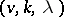-design on a-setA-design is a system of-subsets (blocks) of the setsuch that every-subset of elements ofappears in exactlyblocks. The class of-designs coincides with the class of balanced incomplete block designs (cf. Block design). The name tactical configuration is given to an incidence system in which every set is incident with exactlyelements, while every element is incident with exactlysets. A-design with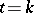is called trivial. If a-design is not trivial, thenEvery-design is an-design for any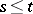. The number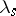of occurrences of an arbitrary-subset in the blocks of a-design is given by the formula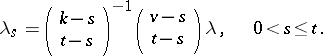The condition that thebe integers is a necessary condition for the existence of a-design. In particular, forevery-design is a balanced incomplete block design.

The main question concerning-designs is the problem of their existence and construction. For a long time, for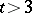only isolated examples were known; in particular-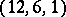- and-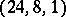-designs connected with the five-fold transitive Mathieu groupsand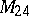(cf. Mathieu group), respectively. However, in the 1960s the connection between-designs and coding theory (cf. Code) was discovered (see, for example,  and ), and, starting from vectors withnon-zero coordinates, a way was shown of constructing a-design belonging to a linear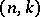-code, which is a-dimensional vector subspace of an-dimensional space over a finite field (see  and ).

It is well known that-fold transitive groups other than the symmetric and the alternating groups lead to non-trivial-designs; this yields a few infinite series of-designs. With the help of ideas from group theory and geometry, infinite classes of- and-designs were also constructed (see for example ).

For the numberof blocks in a-design there is the inequality(*)

generalizing the Fisher inequality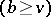for balanced incomplete block designs. In the case of equality in (*), the-design is called tight. Tight-designs generalize symmetric-designs; in particular, forthe set of intersection numbers of blocks in a tight-design contains exactlydifferent elements.

The tight-designs are the Hadamard designs, i.e. they are the-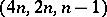-designs, while forthere are no non-trivial tight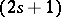-designs. From a given--design three other-designs can be obtained: a) taking the complement inof each block; b) discarding an arbitrary element and all the blocks that contain it; and c) taking blocks containing an arbitrarily chosen element and removing it from them. The-designs thus obtained are called, respectively, the complementary, the residual and the derived-design with respect to the initial one. They are, respectively, a-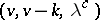-design with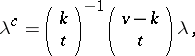a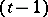-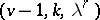-design with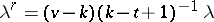and a-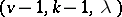-design.

How to Cite This Entry:
Tactical configuration. Encyclopedia of Mathematics. URL: http://encyclopediaofmath.org/index.php?title=Tactical_configuration&oldid=19091
This article was adapted from an original article by V.E. Tarakanov (originator), which appeared in Encyclopedia of Mathematics - ISBN 1402006098. See original article